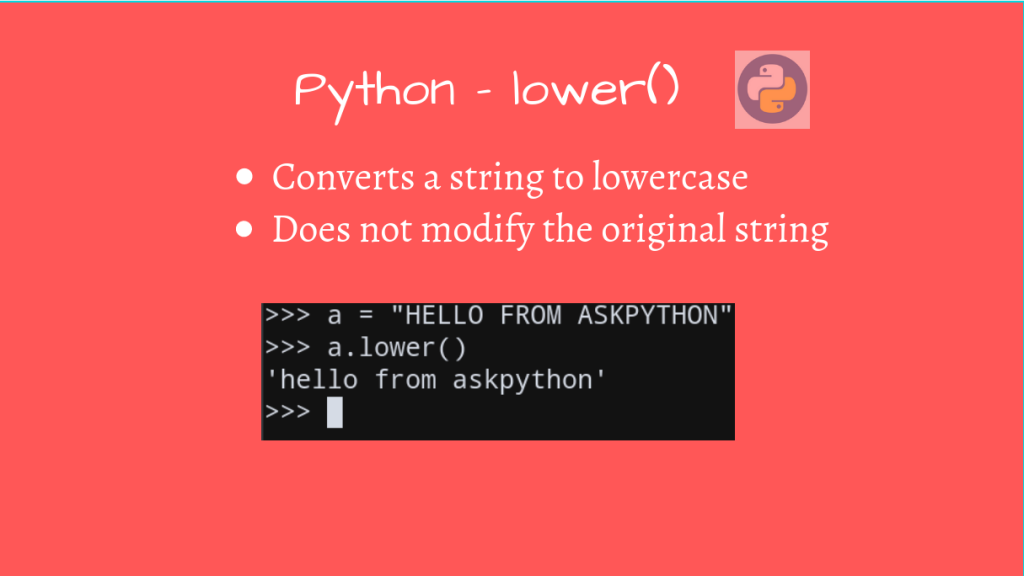# Python String lower() MethodPython String lower() method converts a string object into a lower case string. This is one of the builtin string functions in Python. Since strings are immutable in Python, this method only returns a copy of the original string.

## Syntax and Usage of Python String lower() Method

Format:

```str_copy = str_orig.lower()
```

Here, `str_copy` is the lowercase string of `str_orig`.

```a = "HELLO FROM ASKPYTHON"

b = a.lower()

print(a)
print(b)
```

Output

```HELLO FROM ASKPYTHON
```

This will make the entire output string lowercase, even if only a part of the input string was uppercase.

```a = "Hello from AskPython"
b = a.lower()

print(a)
print(b)
```

Output

```Hello from AskPython
```

Since any string literal is handled as a Unicode by Python3, it can lowercase different languages too.

```>>> string = 'Километр'
>>> string
'Километр'
>>> string.lower()
'километр'
```

## Pandas module – lower()

There is a `lower()` method in the Pandas module as well, which has the same functionality as the native Python method, but is for Pandas Objects.

Format:

```pandas_copy = pandas_object.str.lower()
```

Here is an example illustrating the same:

```>>> import pandas as pd
>>>
>>> s = pd.Series(['Hello', 'from', 'ASKPYTHON'])
>>> print(s)
0        Hello
1         from
dtype: object
>>>
>>> print(s.str.lower())
0        hello
1         from